Open in App
Not now

# Apply a function to each row or column in Dataframe using pandas.apply()

• Difficulty Level : Medium
• Last Updated : 19 Jul, 2021

There are different ways to apply a function to each row or column in DataFrame. We will learn about various ways in this post. Let’s create a small dataframe first and see that.

## Python3

 `# import pandas and numpy library` `import` `pandas as pd` `import` `numpy as np` ` `  `# list of tuples` `matrix ``=` `[(``1``,``2``,``3``,``4``),` `          ``(``5``,``6``,``7``,``8``,),` `          ``(``9``,``10``,``11``,``12``),` `          ``(``13``,``14``,``15``,``16``)` `         ``]` ` `  `# Create a Dataframe object` `df ``=` `pd.DataFrame(matrix, columns ``=` `list``(``'abcd'``))` ` `  `# Output` `df`

Output :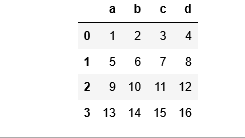Method 1: Applying lambda function to each row/column.
Example 1: For Column

## Python3

 `# import pandas and numpy library` `import` `pandas as pd` `import` `numpy as np`   `# list of tuples` `matrix ``=` `[(``1``,``2``,``3``,``4``),` `          ``(``5``,``6``,``7``,``8``,),` `          ``(``9``,``10``,``11``,``12``),` `          ``(``13``,``14``,``15``,``16``)` `         ``]`   `# Create a Dataframe object` `df ``=` `pd.DataFrame(matrix, columns ``=` `list``(``'abcd'``))`   `# Applying a lambda function to each ` `# column which will add 10 to the value` `new_df ``=` `df.``apply``(``lambda` `x : x ``+` `10``)`   `# Output` `new_df`

Output :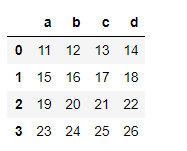Example 2: For Row

## Python3

 `# import pandas and numpy library` `import` `pandas as pd` `import` `numpy as np`   `# list of tuples` `matrix ``=` `[(``1``,``2``,``3``,``4``),` `          ``(``5``,``6``,``7``,``8``,),` `          ``(``9``,``10``,``11``,``12``),` `          ``(``13``,``14``,``15``,``16``)` `         ``]`   `# Creating a Dataframe object` `df ``=` `pd.DataFrame(matrix, columns ``=` `list``(``'abcd'``))`   `# Applying a lambda function to each ` `# row which will add 5 to the value` `new_df ``=` `df.``apply``(``lambda` `x: x ``+` `5``, axis ``=` `1``)`   `# Output` `new_df`

Output :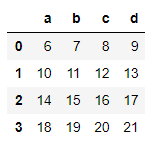Method 2: Applying user defined function to each row/column
Example 1: For Column

## Python3

 `# function to returns x*x` `def` `squareData(x):` `    ``return` `x ``*` `x`   `# import pandas and numpy packages` `import` `pandas as pd` `import` `numpy as np`   `# list of tuples` `matrix ``=` `[(``1``,``2``,``3``,``4``),` `          ``(``5``,``6``,``7``,``8``,),` `          ``(``9``,``10``,``11``,``12``),` `          ``(``13``,``14``,``15``,``16``)` `         ``]`   `# Creating a Dataframe object` `df ``=` `pd.DataFrame(matrix, columns ``=` `list``(``'abcd'``))`   `# Applying a user defined function to ` `# each column that will square the given` `# value` `new_df ``=` `df.``apply``(squareData)`   `# Output` `new_df`

Output :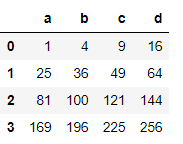Example 2: For Row

## Python3

 `# function to returns x*X` `def` `squareData(x):` `    ``return` `x ``*` `x`   `# import pandas and numpy library` `import` `pandas as pd` `import` `numpy as np`   `# List of tuples` `matrix ``=` `[(``1``,``2``,``3``,``4``),` `          ``(``5``,``6``,``7``,``8``,),` `          ``(``9``,``10``,``11``,``12``),` `          ``(``13``,``14``,``15``,``16``)` `         ``]`   `# Creating a Dataframe object` `df ``=` `pd.DataFrame(matrix, columns ``=` `list``(``'abcd'``))`   `# Applying a user defined function ` `# to each row that will square the given value` `new_df ``=` `df.``apply``(squareData, axis ``=` `1``)`   `# Output` `new_df`

Output :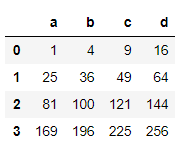In the above examples, we saw how a user defined function is applied to each row and column. We can also apply user defined functions which take two arguments.

Example 1: For Column

## Python3

 `# function to returns x+y` `def` `addData(x, y):` `    ``return` `x ``+` `y`   `# import pandas and numpy library` `import` `pandas as pd` `import` `numpy as np`   `# list of tuples` `matrix ``=` `[(``1``,``2``,``3``,``4``),` `          ``(``5``,``6``,``7``,``8``,),` `          ``(``9``,``10``,``11``,``12``),` `          ``(``13``,``14``,``15``,``16``)` `         ``]`   `# Creating a Dataframe object` `df ``=` `pd.DataFrame(matrix, columns ``=` `list``(``'abcd'``))`   `# Applying a user defined function to each ` `# column which will add value in each` `# column by given number` `new_df ``=` `df.``apply``(addData, args ``=` `[``1``])`   `# Output` `print``(new_df)`

Output: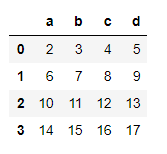Example 2: For Row

## Python3

 `# function to returns x+y` `def` `addData(x, y):` `    ``return` `x ``+` `y`   `# import pandas and numpy library` `import` `pandas as pd` `import` `numpy as np`   `# List of tuples` `matrix ``=` `[(``1``,``2``,``3``,``4``),` `          ``(``5``,``6``,``7``,``8``,),` `          ``(``9``,``10``,``11``,``12``),` `          ``(``13``,``14``,``15``,``16``)` `         ``]`   `# Creating a Dataframe object` `df ``=` `pd.DataFrame(matrix, columns ``=` `list``(``'abcd'``))`   `# Applying a user defined function to each ` `# row which will add value in each row by` `# given number` `new_df ``=` `df.``apply``(addData, axis ``=` `1``,` `                    ``args ``=` `[``3``])`   `# Output` `new_df`

Output :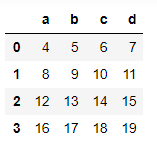Method 3: Applying numpy function to each row/column
Example 1: For Column

## Python3

 `# import pandas and numpy library` `import` `pandas as pd` `import` `numpy as np`   `# list of tuples` `matrix ``=` `[(``1``,``2``,``3``,``4``),` `          ``(``5``,``6``,``7``,``8``,),` `          ``(``9``,``10``,``11``,``12``),` `          ``(``13``,``14``,``15``,``16``)` `         ``]`   `# Creating a dataframe object` `df ``=` `pd.DataFrame(matrix, columns ``=` `list``(``'abcd'``))`   `# Applying a numpy function to each ` `# column by squaring each value ` `new_df ``=` `df.``apply``(np.square)`   `# Output` `new_df`

Output :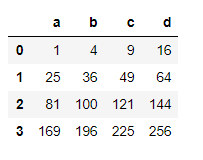Example 2: For Row

## Python3

 `# import pandas and numpy library` `import` `pandas as pd` `import` `numpy as np`   `# List of tuples` `matrix ``=` `[(``1``,``2``,``3``,``4``),` `          ``(``5``,``6``,``7``,``8``,),` `          ``(``9``,``10``,``11``,``12``),` `          ``(``13``,``14``,``15``,``16``)` `         ``]`   `# Creating a dataframe object` `df ``=` `pd.DataFrame(matrix, columns ``=` `list``(``'abcd'``))`   `# Apply a numpy function to each row` `# to find square root of each value ` `new_df ``=` `df.``apply``(np.sqrt, axis ``=` `1``)`   `# Output` `new_df`

Output :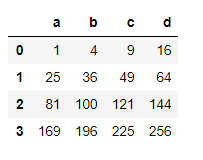Method 4: Applying a Reducing function to each row/column
A Reducing function will take row or column as series and returns either a series of same size as that of input row/column or it will return a single variable depending upon the function we use.

Example 1: For Column

## Python3

 `# import pandas and numpy library` `import` `pandas as pd` `import` `numpy as np`   `# List of tuples` `matrix ``=` `[(``1``,``2``,``3``,``4``),` `          ``(``5``,``6``,``7``,``8``,),` `          ``(``9``,``10``,``11``,``12``),` `          ``(``13``,``14``,``15``,``16``)` `         ``]`   `# Creating a Dataframe object` `df ``=` `pd.DataFrame(matrix, columns ``=` `list``(``'abcd'``))`   `# Applying a numpy function to get the sum ` `# of all values in each column` `new_df ``=` `df.``apply``(np.``sum``)`   `# Output` `new_df`

Output :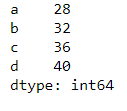Example 2: For Row

## Python3

 `# import pandas and numpy library` `import` `pandas as pd` `import` `numpy as np`   `# List of tuples` `matrix ``=` `[(``1``,``2``,``3``,``4``),` `          ``(``5``,``6``,``7``,``8``,),` `          ``(``9``,``10``,``11``,``12``),` `          ``(``13``,``14``,``15``,``16``)` `         ``]`   `# Creating a Dataframe object` `df ``=` `pd.DataFrame(matrix, columns ``=` `list``(``'abcd'``))`   `# Applying a numpy function to get t` `# he sum of all values in each row` `new_df ``=` `df.``apply``(np.``sum``, axis ``=` `1``)`   `# Output` `new_df`

Output :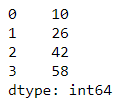My Personal Notes arrow_drop_up
Related Articles